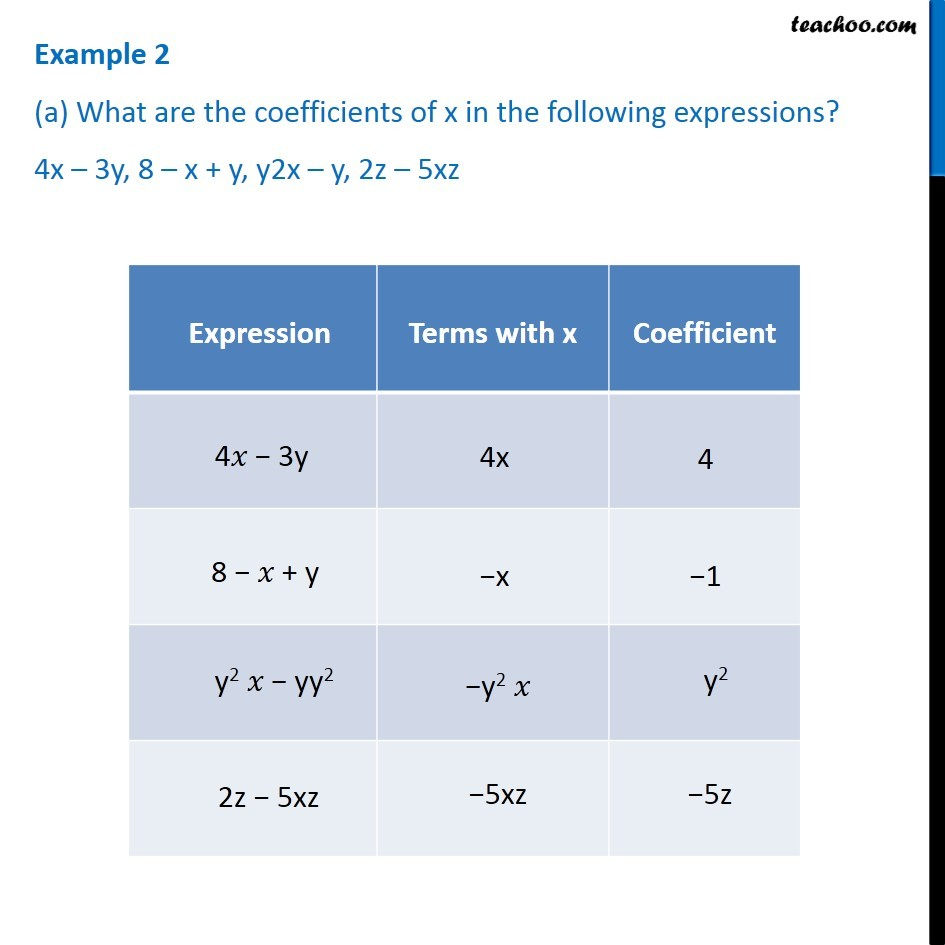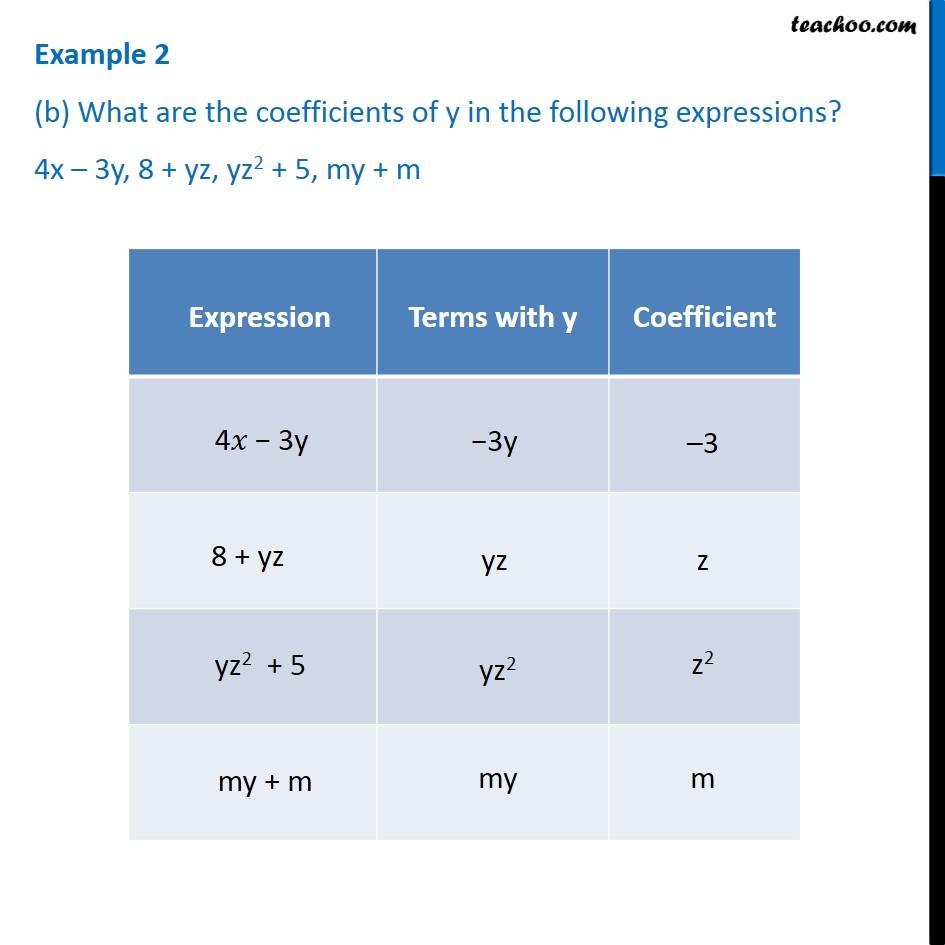Subscribe to our Youtube Channel - https://you.tube/teachoo

1. Chapter 12 Class 7 Algebraic Expressions
2. Serial order wise
3. Examples

Transcript

Example 2 (a) What are the coefficients of x in the following expressions? 4x – 3y, 8 – x + y, y2x – y, 2z – 5xz Expression Terms with x Coefficient 4𝑥 − 3y 4x 4 8 − 𝑥 + y −x −1 y2 𝑥 − yy2 −y2 𝑥 y2 2z − 5xz −5xz −5z Example 2 (b) What are the coefficients of y in the following expressions? 4x – 3y, 8 + yz, yz2 + 5, my + m Expression Terms with x Coefficient 4𝑥 − 3y −3y −3 8 + yz yz z yz2 + 5 −yz2 𝑥 z2 my + m my m

Examples